0

# 你的模型是最好的还是最幸运的？选择最佳模型时如何避免随机性

kaggle比赛里经常会发生shake up的现象，说的直接点就是在有切榜或多榜单的比赛中,可能存在榜单排名激烈震动的情况，例如下面这个例子：Data Science Bowl 2017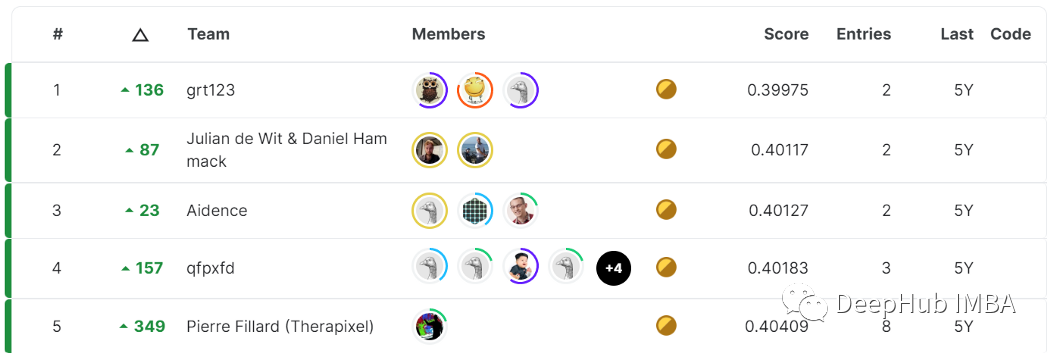## Universe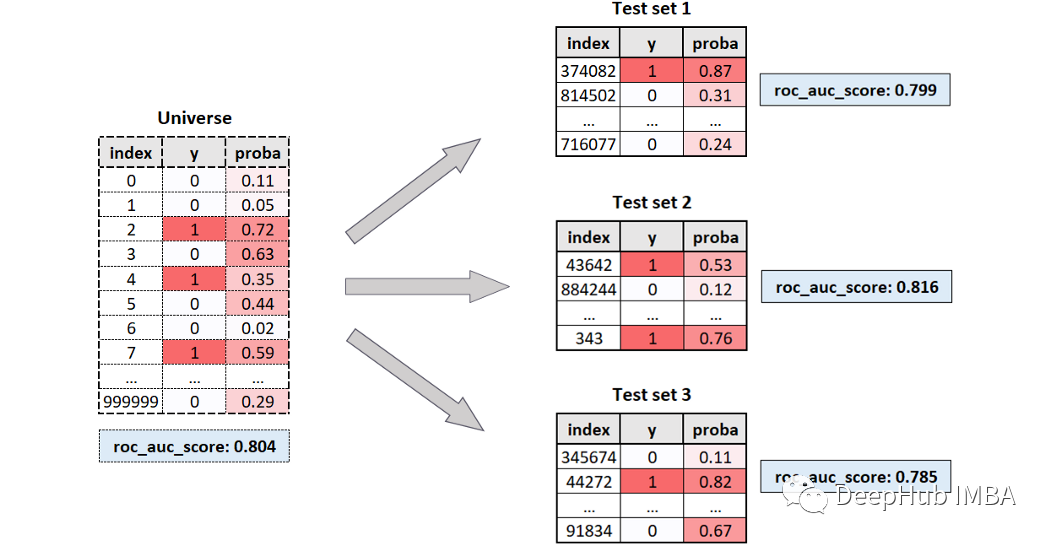## 如何模拟Universe?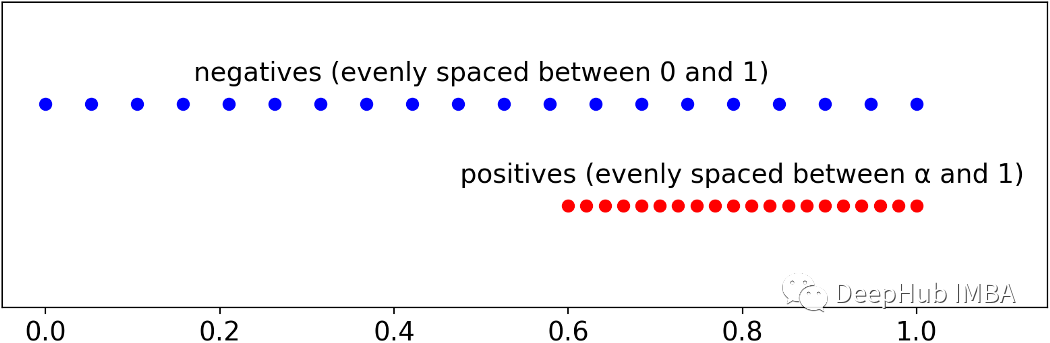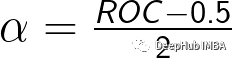`````` def get_y_proba(roc, n=100000, prevalence=.5):
n_ones = int(round(n * prevalence))
n_zeros = n - n_ones
y = np.array( * n_zeros +  * n_ones)
alpha = (roc - .5) * 2

proba_zeros = np.linspace(0, 1, n_zeros)
proba_ones = np.linspace(alpha, 1, n_ones)
proba = np.concatenate([proba_zeros, proba_ones])

return y, proba
``````

## 获取我们的不确定性

`````` y_universe, proba_universe = get_y_proba(roc=.8, n=100000, prevalence=.5)
``````

`````` rocs_sample = []
for i in range(5_000):
index = np.random.choice(range(len(y_universe)), 1_000, replace=True)
y_sample, proba_sample = y[index], proba[index]
roc_sample = roc_auc_score(y_sample, proba_sample)
rocs_sample.append(roc_sample)
``````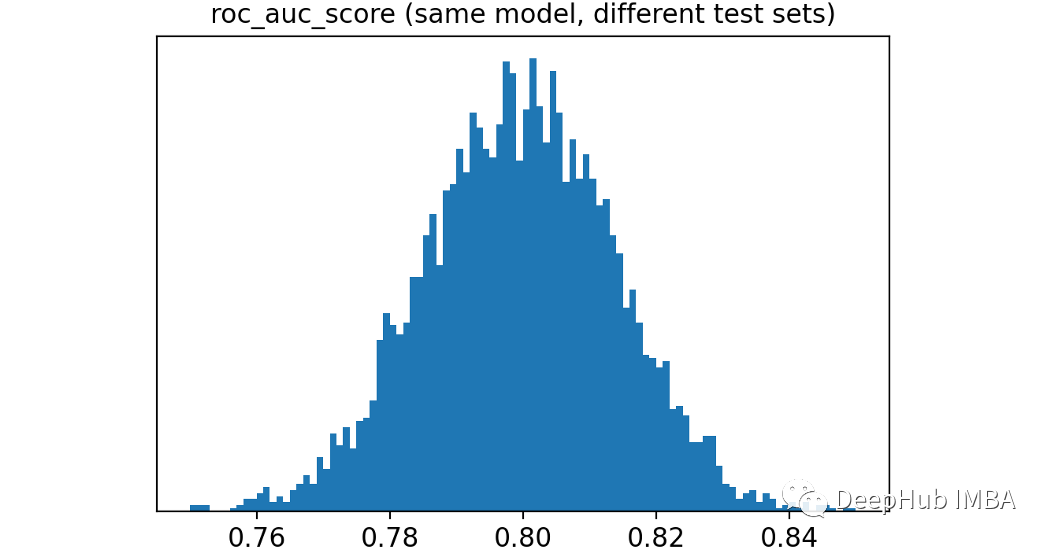`````` import numpy as np
from sklearn.metrics import pairwise_distances

dist = pairwise_distances(np.array(rocs_sample).reshape(-1,1))
dist = dist[np.triu_indices(len(rocs_sample), k=1)]
``````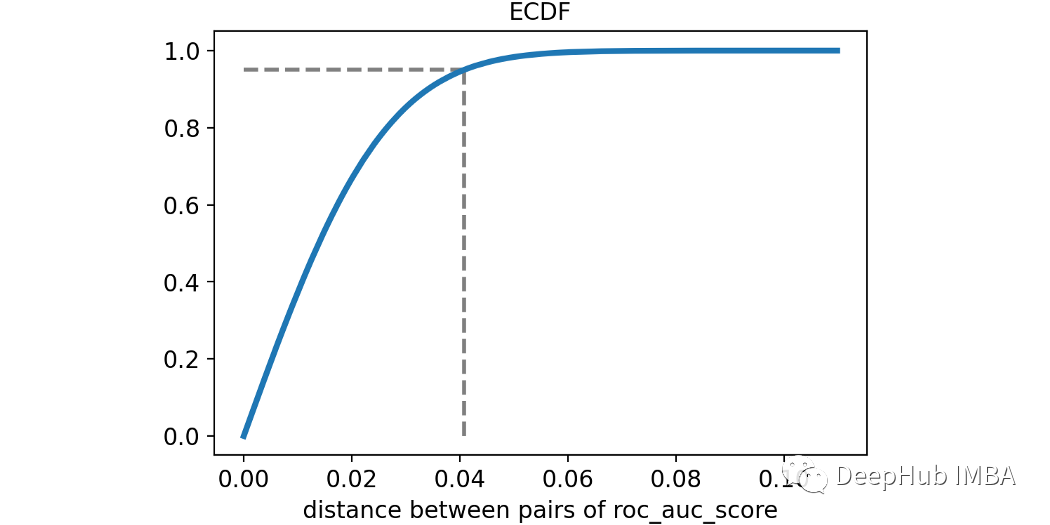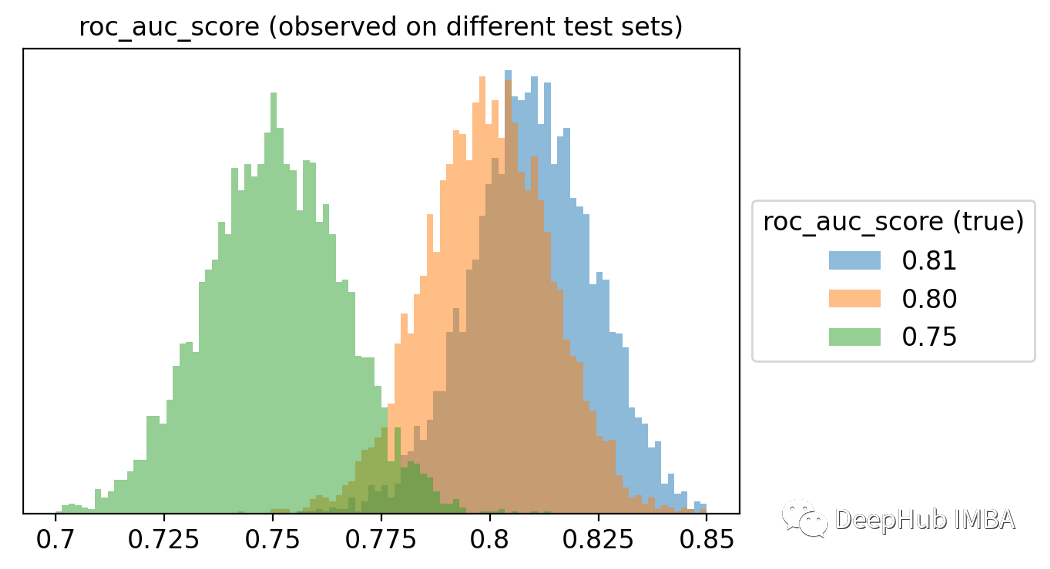## 还能做点什么吗?

• 真实ROC:在universe中计算的ROC得分（这个肯定是得不到的，所以只能假设）。
• 样本数:测试集中的样本总数。
• 样本流行率（prevalence）:测试集中的阳性百分比。

• 真实ROC: 70%， 80%和90%。
• 样本数:1000，5000和10000。
• 样本流行率:1%，5%和20%。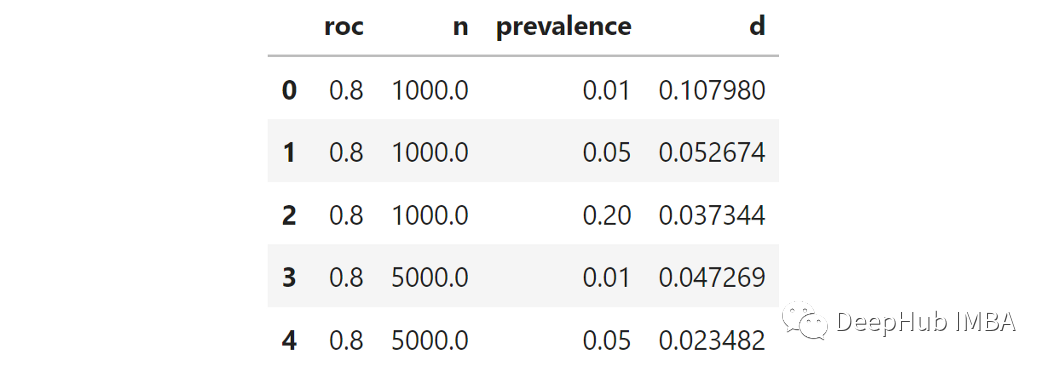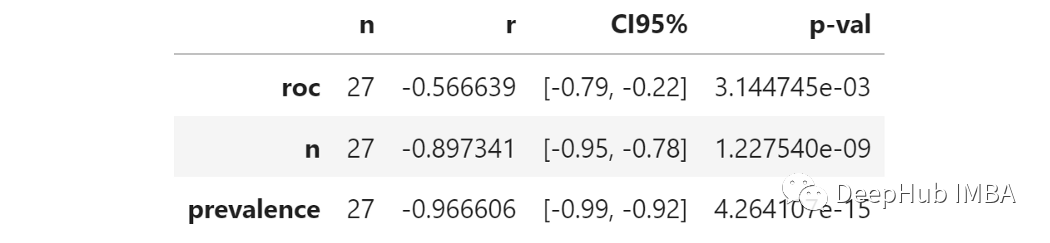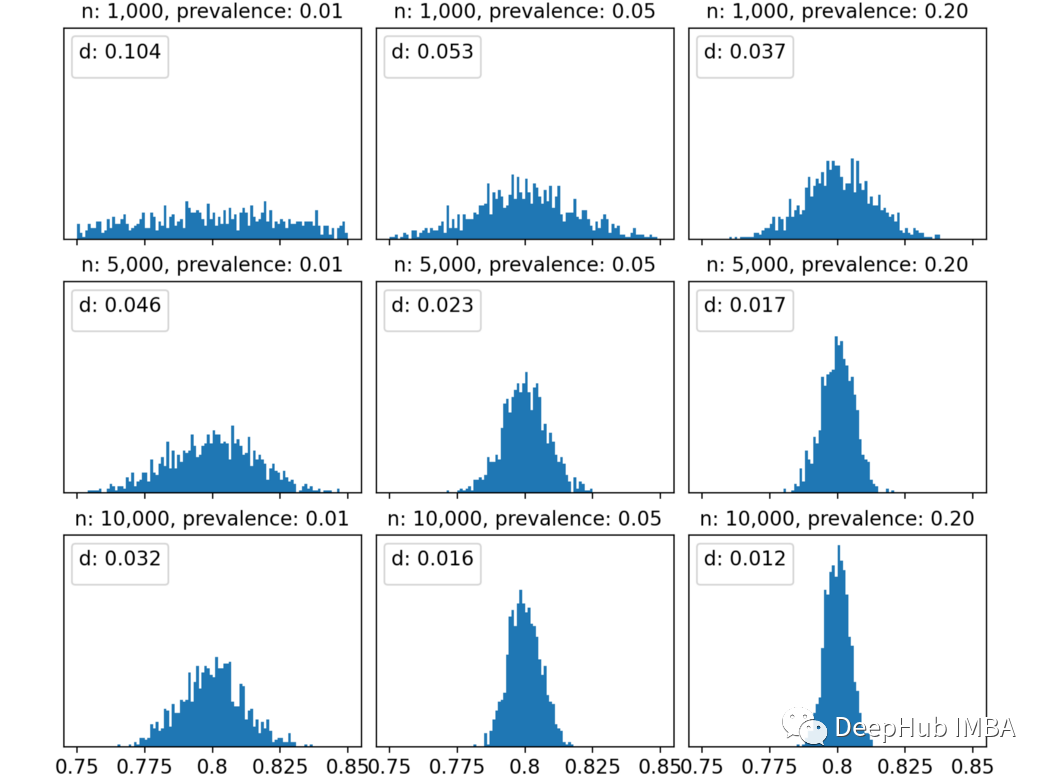## 这对我有用吗？

### “你的模型是最好的还是最幸运的？选择最佳模型时如何避免随机性”的评论:

##### 关于作者##### Deephub